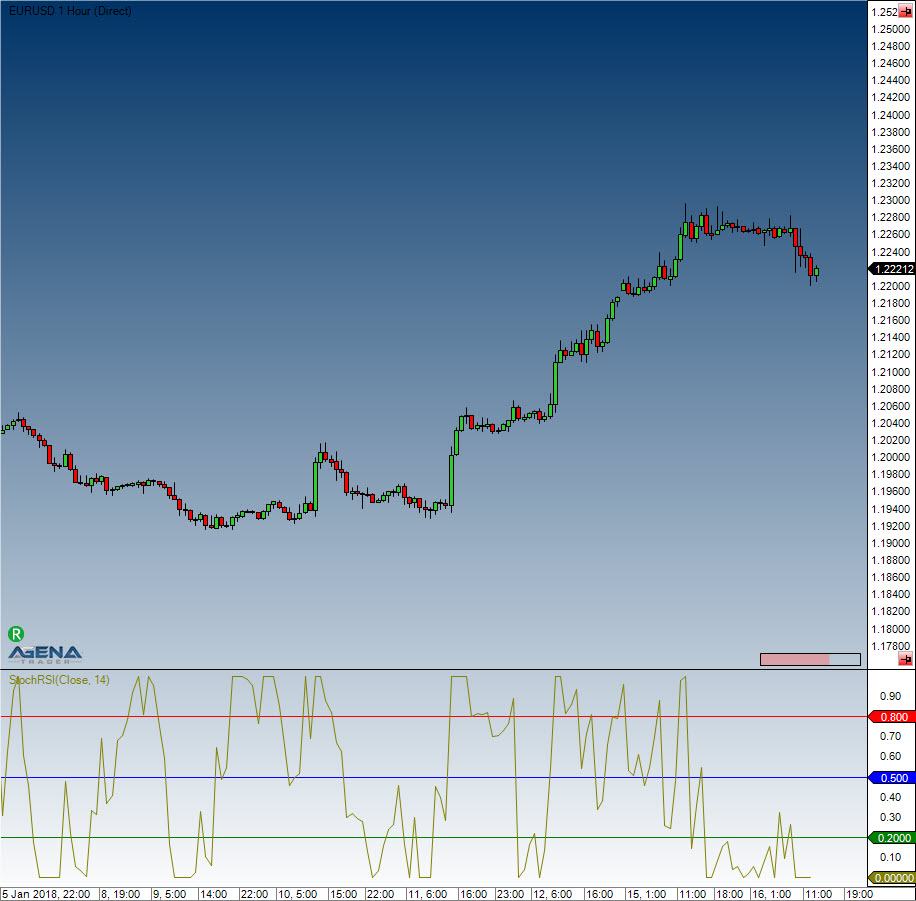# Stochastics RSI (StochRSI)

#### Description

Stochastics RSI is a calculation of the stochastic based on the RSI indicator.

#### Interpretation

The indicator provides fast and precise extreme values/points in the price movements. The StochRSI can be used as a trend filter in higher timeframes as well as a tool for better entry timing.

#### Usage

StochRSI(int period)
StochRSI(IDataSeries inSeries, int period)
StochRSI(int period)[int barsAgo]
StochRSI(IDataSeries inSeries, int period)[int barsAgo]

#### Return value

double
When using this method with an index (e.g. StochRSI(14)[int barsAgo] ), the value of the indicator will be issued for the referenced bar.

#### Parameters

inSeries Input data series for the indicator
period Number of bars included in the calculations

#### VisualizationStochastics RSI (StochRSI)

#### Example

//Output for the StochRSI
Print("The current value for the StochRSI is: " + StochRSI(14));